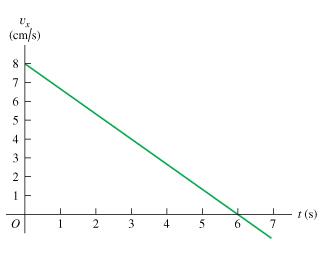# Problem: A cat walks in a straight line, which we shall call the x-axis with the positive direction to the right. As an observant physicist, you make measurements of this cats motion and construct a graph of the felines velocity as a function of time (the figure ).(a) Find the cats velocity at exttip{t}{t1} = 5.0 s .(b) What is the cats acceleration at t = 3.0?(c) What distance does the cat move during the first 4.5 s?(d) Sketch clear graph of the cats acceleration as function of time, assuming that the cat started at the origin.(e) Find the cats velocity at exttip{t}{t2} = 8.0 s .(f) What is the cats acceleration at t = 6.0?(g) What is the cats acceleration at t = 7.0?(h) What total distance does the cat move from t = 0 to t = 7.5?(i) Sketch clear graph of the cats position as function of time, assuming that the cat started at the origin.

###### FREE Expert Solution

In this problem, we are given a velocity-time graph. We'll deduce various information from the graph to answer the following questions.

(a)

In velocity time-graphs, the velocity at 5.0s is read from the graph as:

• Draw a vertical line passing through t = 5.0s to touch the curve.
• Draw a horizontal line to touch the same point on the curve. Read the value of the velocity.
97% (355 ratings)###### Problem Details

A cat walks in a straight line, which we shall call the x-axis with the positive direction to the right. As an observant physicist, you make measurements of this cats motion and construct a graph of the felines velocity as a function of time (the figure).

(a) Find the cats velocity at = 5.0 s .

(b) What is the cats acceleration at t = 3.0?

(c) What distance does the cat move during the first 4.5 s?

(d) Sketch clear graph of the cats acceleration as function of time, assuming that the cat started at the origin.

(e) Find the cats velocity at = 8.0 s .

(f) What is the cats acceleration at t = 6.0?

(g) What is the cats acceleration at t = 7.0?

(h) What total distance does the cat move from t = 0 to t = 7.5?

(i) Sketch clear graph of the cats position as function of time, assuming that the cat started at the origin.

Frequently Asked Questions

What scientific concept do you need to know in order to solve this problem?

Our tutors have indicated that to solve this problem you will need to apply the Graphing Position, Velocity, and Acceleration Graphs concept. You can view video lessons to learn Graphing Position, Velocity, and Acceleration Graphs. Or if you need more Graphing Position, Velocity, and Acceleration Graphs practice, you can also practice Graphing Position, Velocity, and Acceleration Graphs practice problems.

What professor is this problem relevant for?

Based on our data, we think this problem is relevant for Professor Moussa's class at UCF.